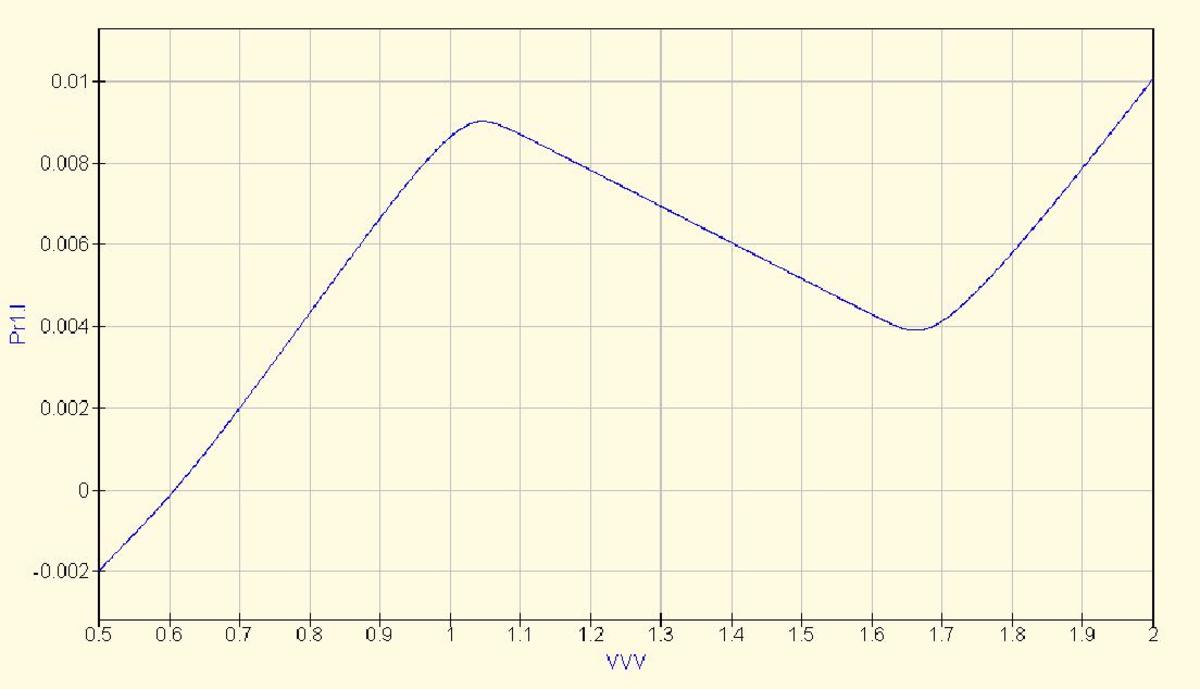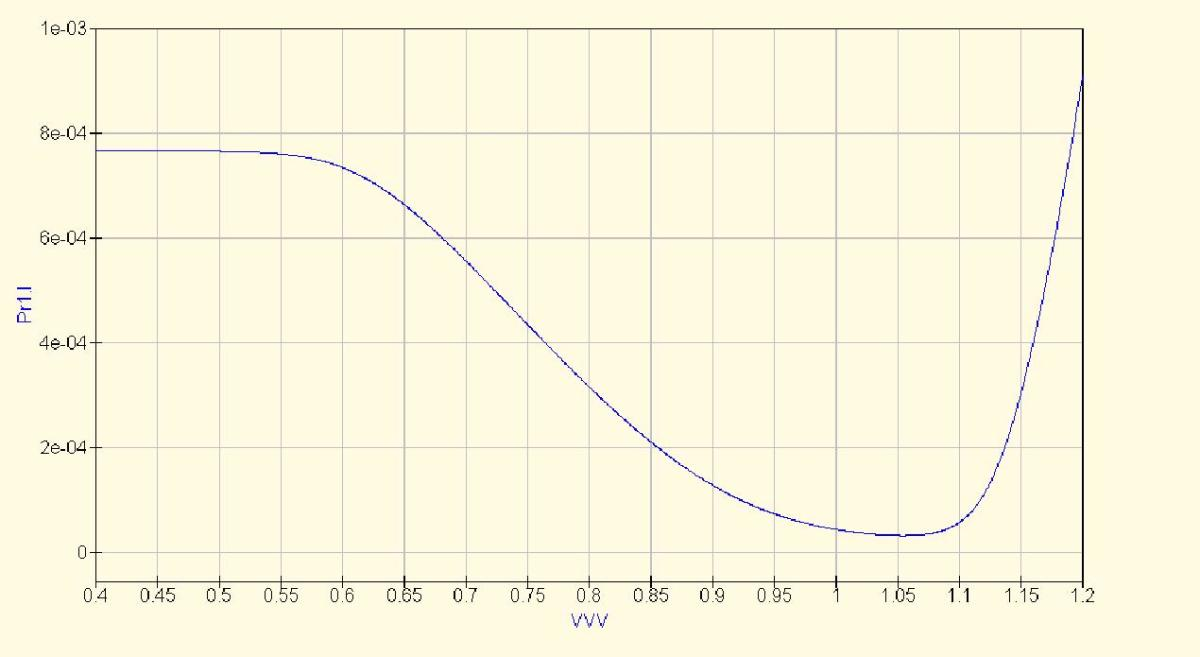Updated date:

# Negative Dynamic Resistance Circuit

## Negative dynamic resistance

With a normal resistor the current flowing through the resistor increases with voltage. With a negative dynamic resistor the current decreases with increasing voltage at least over some portion of the input voltage range. Figure 1 shows a little known negative dynamic resistance circuit. As voltage V2 increases the current at first increases as per a normal resistor. However at slightly over 1 volt the current starts to fall in a very linear fashion with increasing voltage (see figure 2) until you reach 1.7 volts where the negative resistance region ends.

## Figure 2Negative dynamic resistance region. The current in Amps is shown on the vertical axes. The voltage V2 is shown on the horizontal axes.

## Simplifying application of the circuit

A slight disadvantage of the circuit in figure 1 is that you must supply an additional voltage V1. If you are willing to accept a lower degree of linearity (figure 4) and a reduced frequency response then you can run the entire circuit using a supply voltage of about 0.7 volts. This is easily obtained by forward biasing a standard silicon diode and results in the circuit show in figure 3. You can experiment with different diodes to put the supply voltage in the middle of the negative resistance range.

## Figure 4The negative resistance curve of the simplified circuit. Notice that the negative resistance region for V2 is in the same range as the forward bias voltage of D1. This means you don't need an extra voltage source.